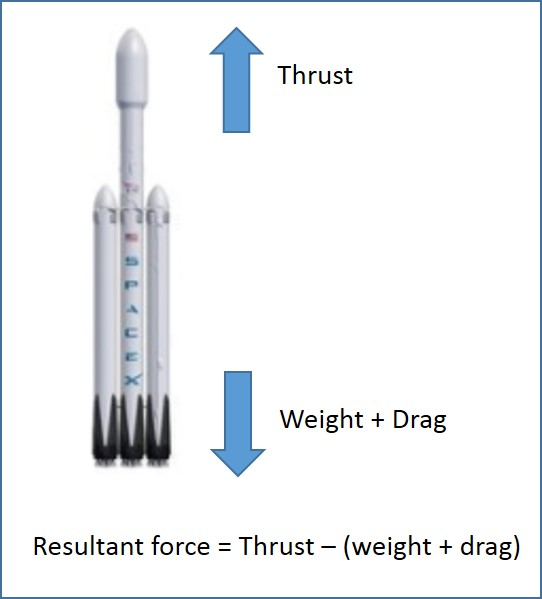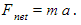# Newton’s Laws Of Motion

## Introduction

Newton’s laws of motion are three physical laws that describe the relationship between a body and the forces acting upon it, and its motion in response to those forces. In general, the first law defines the force qualitatively, the second law offers a quantitative measure of the force, and the third asserts that a single isolated force doesn’t exist. In this article, Newton’s laws of motion and their practical implications are explained.

A. Newton’s First Law

Newton’s first law states that an object will remain at rest or in uniform motion in a straight line unless acted upon by an external force.

Where it Applies

Consider a wagon as illustrated in Figure 1. In the absence of an external force, the wagon will not move. When pulling the wagon with some amount of force (F), it accelerates and starts to move. Thus, Newton’s first law can be demonstrated. Newton’s first law can also be applied in places such as:

1. An aircraft flying at a constant speed will remain so until an external force acts on it to stop.
2. A football lying on a field will remain idle until someone kicks it.Figure 1: Newton’s First Law

B. Newton’s Second Law

Newton’s second law states that the acceleration of an object as produced by a net force is directly proportional to the magnitude of the net force, in the same direction as the net force, and inversely proportional to the mass of the object.Where it Applies

Consider two wagons, as illustrated in Figure 2, where the weight placed on the second wagon (m2) is higher than the weight placed on the first wagon (m1). When pulling these two wagons with the same amount of force, acceleration in the second wagon (a2) will be less than that of the first wagon (a1). This shows that net force is inversely proportional to the mass of the object.Figure 2: Newton’s Second Law

C. Newton’s Third Law

Newton’s third law states that for every action, there is an equal and opposite reaction. The law means that in every interaction, there is a pair of forces acting on the two interacting objects.

Where it Applies

In a vertical launch of a rocket (Figure 3), the weight of the rocket and drag (air resistance) act downwards. The thrust from the propulsion of the rocket acts upwards. When the thrust force is higher than the combined forces of weight and drag, then the rocket moves upwards.Figure 3: Newton’s Third Law

Summary

• Newton’s first law states that an object will remain at rest or in uniform motion in a straight line unless acted upon by an external force.
• Newton’s second law states that the acceleration of an object as produced by a net force is directly proportional to the magnitude of the net force, in the same direction as the net force, and inversely proportional to the mass of the object. In equation form, Newton’s second law can be expressed as.
• Newton’s third law states that for every action, there is an equal and opposite reaction.